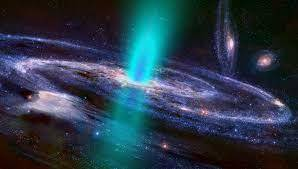Hubble's lawHubble'

s law states that "the speed of recession of a galaxy is proportional to its distance from us."Hence, If v is the speed with which a galaxy recedes and r is its distance from the earth,

Then,

v∝r

or, v = H0r

where, H0﻿ is called Hubble's constant. It's value is 2.3*10-18﻿ s-1﻿.

Significance of Hubble's law:

It is used to predict the age of the universe.

According to Hubble's law, matter at a distance r away from us is travelling with speed v=H0r.

The time t needed to travel a distance r is given by, t=r/v

so, t=r/H0r

=1/H0

= 4.3 * 10^17 s

= 1.4 *10^10 years

Hence we can conclude that the universe is 1.4 *10^10 years old.